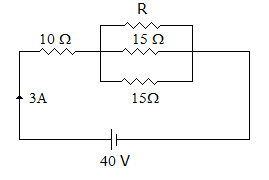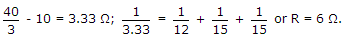# Electronics and Communication Engineering - Networks Analysis and Synthesis

1.

In figure, the value of R should beA. 12 Ω B. 6 Ω C. 3 Ω D. 1.5 Ω

Explanation:

The resistance of parallel combination =2.

An RLC series circuit has R = 7.07 Ω, L = .707 H and C = 7.07 μF. At Half power frequencies the circuit impedance is likely to be

 A. 7.07 Ω B. 10 Ω C. 14.14 Ω D. 20 Ω

Explanation:

At half power frequency the circuit impedance is 2 times resistance.

3.

In a series R, L, C circuit the impedance triangle is the same when XL < XC and XL > XC .

 A. True B. False

Explanation:

4.

The zinc can of a dry cell serves as positive terminal.

 A. True B. False

Explanation:

Zinc can serves as negative terminal.

5.

Two similar coils have self inductance of 1 mH each. Coefficient of coupling is 0.5. The mutual inductance M is

 A. 0.25 mH B. 0.5 mH C. 0.707 mH D. 1 mH